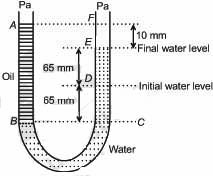## Filters

Sort by :
Clear All
Q
Medical
477 Views   |

The bulk modulus of a spherical object is 'B'. If it is subjected to uniform to uniform pressure 'p', the fractional decrease in radius is:

• Option 1)

• Option 2)

• Option 3)

• Option 4)

Bulk Modulus - Ratio of normal stress to volumetric strain. v = Original  volume  = Change in volume P = Increase in pressure -ve(sign) shows volume  decrease. - wherein      or  Put this value in equation (i) we get fractional decrease in radius is Option 1) This solution is incorrect Option 2) This solution is incorrect Option 3) This solution is correct Option 4) This solution...
Medical
845 Views   |

A U tube with both ends open to the atmosphere, is partially filled with water. Oil, which is immiscible with water, is poured into one side until it stands at a distance of 10 mm above the water level on the other side. Meanwhile the water rises by 65 mm from its original level (see diagram). The density of the oil is• Option 1)

650 kg m-3

• Option 2)

425 kg m-3

• Option 3)

800 kg m-3

• Option 4)

928 kg m-3

@7561r Gauge pressure - Pressure diffrence  between hydrostatic and atmospheric pressure - wherein      Pressure of two points lie in the same horizontal level should be same and  Both ends of tube is open, so the pressure on both the free surface must be equal.     Option 1) 650 kg m-3 This option is incorrect. Option 2) 425 kg m-3 This option is incorrect. Option 3) 800 kg m-3 This...
Medical
777 Views   |

A spherical black body with a radius of 12 cm radiates 450 watt power at 500 K. If the radius were halved and the temperature doubled, the power radiated in watt would be :

• Option 1)

225

• Option 2)

450

• Option 3)

1000

• Option 4)

1800

As we learnt in Radiant Power (P) - -    Power of radiation is      (For blackbody) According to question Then  or P2 = 4P1 = 4  450 W = 1800 W Correct option is 4.   Option 1) 225 Incorrect Option 2) 450 Incorrect Option 3) 1000 Incorrect Option 4) 1800 Correct
Exams
Articles
Questions